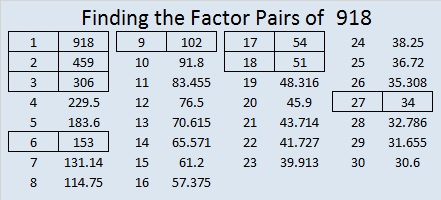# 918 Grim Reaper’s Scythe

Sometime on Halloween you are likely to see the Grim Reaper carrying a scythe. Together they look pretty scary. This puzzle isn’t that bad though. You should give it a try.Print the puzzles or type the solution on this excel file: 10-factors-914-922

Scythe, now that is a good word to try when playing hangman. ☺

Let me tell you about the number 918:

It is the sum of consecutive prime numbers: 457 + 461 = 918

It is the hypotenuse of a Pythagorean triple:
432-810-918, which is (8-15-17) times 54

918 looks interesting in a few other bases:

• 646 in BASE 12, because 9(144) + 4(12) + 6(1) = 918
• 330 in BASE 17, because 3(289) + 3(17) + 0(1) = 3(289 + 17) = 3(306) = 918
• 198 in BASE 26, which is the digits of 918 in a different order. Note that 1(26²) + 9(26) + 8(1) = 918
• RR in BASE 33, (R is 27 in base 10), because 27(33) + 27(1) = 27(33 + 1) = 27(34) = 918
• R0 in BASE 34, because 27(34) = 918

918 has consecutive numbers, 17 and 18, as two of its factors. That means 918 is a multiple of the 17th triangular number, 153.

• 918 is a composite number.
• Prime factorization: 918 = 2 × 3 × 3 × 3 × 17, which can be written 918 = 2 × 3³ × 17
• The exponents in the prime factorization are 1, 3, and 1. Adding one to each and multiplying we get (1 + 1)(3 + 1)(1 + 1) = 2 × 4 × 2 = 16. Therefore 918 has exactly 16 factors.
• Factors of 918: 1, 2, 3, 6, 9, 17, 18, 27, 34, 51, 54, 102, 153, 306, 459, 918
• Factor pairs: 918 = 1 × 918, 2 × 459, 3 × 306, 6 × 153, 9 × 102, 17 × 54, 18 × 51, or 27 × 34
• Taking the factor pair with the largest square number factor, we get √918 = (√9)(√102) = 3√102 ≈ 30.29851This site uses Akismet to reduce spam. Learn how your comment data is processed.